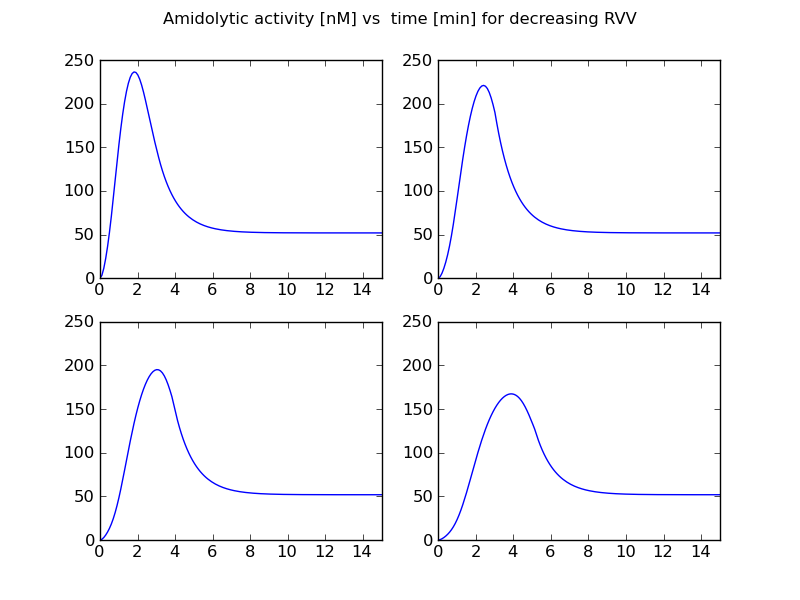## Stortelder1997 - Thrombin Generation Amidolytic ActivityModel Identifier
BIOMD0000000358
Short description
Stortelder1997 - Thrombin Generation Amidolytic Activity

Mathematical modelling of a part of the blood coagulation mechanism.

This model is described in the article:

Stortelder W.J.H., Hemker P.W., Hemker, H.C.
CWI. Modelling, Analysis and Simulation, No. R 9720, p.1-11.

Abstract:

This paper describes the mathematical modelling of a part of the blood coagulation mechanism. The model includes the activation of factor X by a purified enzyme from Russel's Viper Venom (RVV), factor V and prothrombin, and also comprises the inactivation of the products formed. In this study we assume that in principle the mechanism of the process is known. However, the exact structure of the mechanism is unknown, and the process still can be described by different mathematical models. These models are put to test by measuring their capacity to explain the course of thrombin generation as observed in plasma after recalcification in presence of RVV. The mechanism studied is mathematically modelled as a system of differential-algebraic equations (DAEs). Each candidate model contains some freedom, which is expressed in the model equations by the presence of unknown parameters. For example, reaction constants or initial concentrations are unknown. The goal of parameter estimation is to determine these unknown parameters in such a way that the theoretical (i.e., computed) results fit the experimental data within measurement accuracy and to judge which modifications of the chemical reaction scheme allow the best fit. We present results on model discrimination and estimation of reaction constants, which are hard to obtain in another way.

To the extent possible under law, all copyright and related or neighbouring rights to this encoded model have been dedicated to the public domain worldwide. Please refer to CC0 Public Domain Dedication for more information.

Format
SBML (L2V4)
Related Publication
• Mathematical modelling in blood coagulation ; Simulation and parameter estimation• Stortelder W.J.H., Hemker P.W., Hemker H.C.
• CWI. Modelling, Analysis and Simulation [MAS], No. R 9720, p.1-11 1997 , Other Link (URL): http://www.narcis.nl/publication/RecordID/oai:cwi.nl:4725
• This paper describes the mathematical modelling of a part of the blood coagulation mechanism. The model includes the activation of factor X by a purified enzyme from Russel's Viper Venom (RVV), factor V and prothrombin, and also comprises the inactivation of the products formed. In this study we assume that in principle the mechanism of the process is known. However, the exact structure of the mechanism is unknown, and the process still can be described by different mathematical models. These models are put to test by measuring their capacity to explain the course of thrombin generation as observed in plasma after recalcification in presence of RVV. The mechanism studied is mathematically modelled as a system of differential-algebraic equations (DAEs). Each candidate model contains some freedom, which is expressed in the model equations by the presence of unknown parameters. For example, reaction constants or initial concentrations are unknown. The goal of parameter estimation is to determine these unknown parameters in such a way that the theoretical (i.e., computed) results fit the experimental data within measurement accuracy and to judge which modifications of the chemical reaction scheme allow the best fit. We present results on model discrimination and estimation of reaction constants, which are hard to obtain in another way.
Contributors
Michael Schubert

is
BioModels Database MODEL1108260009
BioModels Database BIOMD0000000358
Gene Ontology blood coagulation
hasTaxon
Taxonomy Eukaryota
isDescribedBy

Curation status
Curated

Tags
Name Description Size Actions

### Model files

BIOMD0000000358_url.xml SBML L2V4 representation of Stortelder1997 - Thrombin Generation Amidolytic Activity 21.26 KB Preview | Download

• Model originally submitted by : Michael Schubert
• Submitted: 26-Aug-2011 17:19:20
##### Revisions
Legends
: Variable used inside SBML models

Species
Species Initial Concentration/Amount
Xa

Coagulation factor X
0.0 nmol
PL 9.999997 nmol
Va

Coagulation factor V
0.0 nmol
IIa

Prothrombin
0.0 nmol
II

Prothrombin
509.2998 nmol
IIa alpha2M

Alpha-2-macroglobulin ; Prothrombin
0.0 nmol
X

Coagulation factor X
81.24998 nmol
Xa ATIII

Antithrombin-III ; Coagulation factor X
0.0 nmol
PT 0.0 nmol
Reactions
Reactions Rate Parameters
compartment_1*kcat_X*RVV*X/(km_X+X)

compartment_1*kcat_X*RVV*X/(km_X+X)
kcat_X = 239.1; km_X = 23.65
compartment_1*(k_PT*Va*Xa*PL-k_PL*PT)

compartment_1*(k_PT*Va*Xa*PL-k_PL*PT)
k_PL = 801.4; k_PT = 122.9
compartment_1*kcat_2*Xa*II/(km_2+II)

compartment_1*kcat_2*Xa*II/(km_2+II)
kcat_2 = 12.4; km_2 = 0.06148
compartment_1*ki_IIaATIII*IIa

compartment_1*ki_IIaATIII*IIa
ki_IIaATIII = 0.7859
compartment_1*kcat_II*PT*II/(km_II+II)

compartment_1*kcat_II*PT*II/(km_II+II)
km_II = 62.25; kcat_II = 43.87
compartment_1*ki_IIaAlpha2M*IIa

compartment_1*ki_IIaAlpha2M*IIa
ki_IIaAlpha2M = 0.1762
compartment_1*ki_Xa*Xa

compartment_1*ki_Xa*Xa
ki_Xa = 4.531
compartment_1*kcat_V*IIa*V/(km_V+V)

compartment_1*kcat_V*IIa*V/(km_V+V)
km_V = 149.7; kcat_V = 7.844Curator's comment:
(added: 26 Aug 2011, 17:23:37, updated: 26 Aug 2011, 17:23:37)
Reproduction of figure 5 of the article. Model integrated using PySCeS and plotted with Matplotlib.